## ML Aggarwal Class 8 Solutions for ICSE Maths Chapter 9 Direct and Inverse Variation Ex 9.1

Question 1.
Observe the following tables and find if x and y are directly proportional:
(i)

 x 5 8 12 15 18 20 y 15 24 36 60 72 100

(ii)

 x 3 5 7 9 10 y 9 15 21 27 30

Solution:
(i) x and y are in direct variation of $$\frac{x}{y}$$ is constant$$\frac{x}{y}$$ is not constantThere are not directly proportional

(ii) $$\frac{x}{y}=\frac{3}{9}=\frac{1}{3}$$
Similarly, $$\frac{5}{15}=\frac{1}{3}, \frac{7}{21}=\frac{1}{3}, \frac{9}{27}=\frac{1}{3}$$ and
$$\frac{10}{30}=\frac{1}{3}$$
∴ $$\frac{x}{y}$$ is constant
Here, x and y are directly proportional.

Question 2.
If x and y are in direct variation, complete the following tables:
(i)

 x 3 5 … 10 y 45 … 90 120

(ii)

 x 4 8 … 20 28 y 7 … 21 … …

Solution: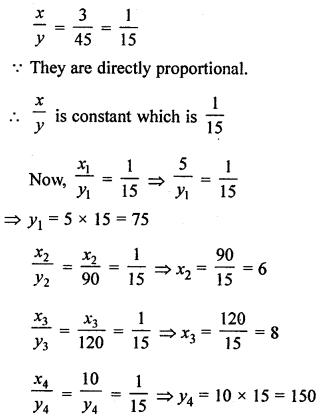Hence,

 x 3 5 6 8 10 y 45 75 90 120 150

(ii)

 x 4 8 … 20 28 y 7 … 21 … …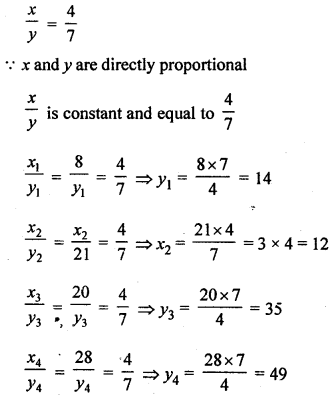Hence

 x 4 8 12 20 28 y 7 14 21 35 49

Question 3.
If 8 metres cloth costs ₹250, find the cost of 5·8 metres of the same cloth.
Solution:
Let the cost of 5·8 m cloth = ₹ x

 Length (in m) 8 5.8 Cost of cloth (₹) 250 x

Hence, it is the case of direct variation
∴ 8 : 250 = 5.8 : x
⇒ $$\frac{8}{250}=\frac{5.8}{x}$$
⇒ $$x=\frac{5.8 \times 250}{8}$$ = ₹181.25

Question 4.
If a labourer earns ₹672 per week, how much will he earn in 18 days?
Solution:
Let the labourer earns ₹ x in 18 days

 Days 7 18 Money earned (in ₹) 672 x

Hence, it is in the case of direct variation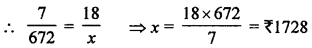Question 5.
If 175 dollars cost ₹7350, how many dollars can be purchased in ₹24024?
Solution:
Let x dollars be purchased in ₹24024

 Cost (in ₹) 7350 24024 Dollars 175 x

Hence, the question is of direct variationQuestion 6.
If a car travels 67·5 km in 4·5 litres of petrol, how many kilometres will it travel in 26.4 litres of petrol?
Solution:
Let car travels x km in 26·4 litres of petrol

 Distance (in km) x 67.5 Petrol (in l) 26.4 4.5

Hence, the question is of direct variation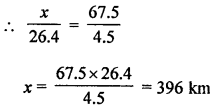Question 7.
If the thickness of a pile of 12 cardboard sheets is 45 mm, then how many sheets of the same cardboard would be 90 cm thick?
Solution:
Thickness of 12 cardboard = 45 mm
Let x be the number of cardboard whose thickness is 90 cm = 900 m
12 : x = 45 : 900 ⇒ $$\frac{12}{x}=\frac{45}{900}$$
⇒ x = $$\frac{12 \times 900}{45}$$ = 240 mm
Thickness of 90 cardboard = 240 mm

Question 8.
In a model of a ship, the mast (flagstaff) is 6 cm high, while the mast of the actual ship is 9 m high. If the length of the ship is 33 m, how long is the model of the ship?
Solution:
Height of a model of ship = 6 cm
But height of actual ship = 9 m
If length of ship = 33 m
Let length of model = x
∴ 6 : x = 9 m : 33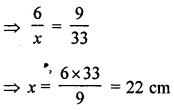∴ Length of model = 22 cm

Question 9.
The mass of an aluminium rod varies directly with its length. If a 16 cm long rod has a mass of 192 g, find the length of the rod whose mass is 105 g.
Solution:
Length of rod = 16 cm and mass = 192 g
If mass is 105 g, then
Let length of rod = x cm
∴ 16 : x = 192 : 105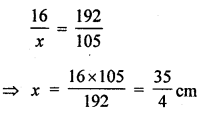= 8.75 cm

Question 10.
Anita has to drive from village A to village B. She measures a distance of 3.5 cm between these villages on the map. What is the actual distance between the villages if the map scale is 1 cm = 20 km?
Solution:
Distance from village A to B on the map = 3.5 cm
Scale of map 1 cm = 20 km
Let actual distance = x km
∴ 1 : 3.5 = 20 : x
$$\frac{1}{3.5}=\frac{20}{x}$$ ⇒ x = 20 × 3.5 km 3.5
⇒ x = 70 km
∴ Distance between village A and village B 20 km

Question 11.
A 23 m 75 cm high water tank casts a shadow 20 m long. Find at the same time;
(i) the length of the shadow cast by a tree 9 m 50 cm high.
(ii) the height of the tree if the length of the shadow is 12 m.
Solution:
Height of a water tank = 23 m 75 cm
= $$23 \frac{3}{4} m=\frac{95}{4} m$$
and its shadow = 20 m
(i) If height of a tree = 9 m 50 cm = $$9 \frac{1}{2}$$ m∴ Its shadow = 8 m
(ii) Let height of a tree = x m
and its shadow = 12 m⇒ x =14 m 25 cm
∴ Height of the tree = 14 m 25 cm

Question 12.
If 5 men or 7 women can earn ₹525 per day, how much would 10 men and 13 women will earn per day.
Solution:
Since in a day, 5 men can earn ₹525
∴ In a day, 1 men can earn = ₹ $$\frac{525}{5}$$ = ₹105
∴ In a day, 10 men will earn ₹105 × 10 = ₹1050
As in a day 7 women can earn ₹525
∴ In a day, 1 women can earn = ₹$$\frac{525}{7}$$ = ₹75
In a day 13 women will earn ₹75 × 13 = ₹975
∴ Total earning of 7 men and 13 women in a day = ₹(1050 + 975) = ₹2025